# 5.4.5 - Logistic Regression Model Diagnostics - Teradata Warehouse Miner

## Teradata Warehouse Miner User Guide - Volume 3Analytic Functions

Product
Release Number
5.4.5
Published
February 2018
Language
English (United States)
Last Update
2018-05-04
dita:mapPath
yuy1504291362546.ditamap
dita:ditavalPath
ft:empty

Logistic regression has counterparts to many of the same model diagnostics available with linear regression. In a similar manner to linear regression, these diagnostics provide a mathematically sound way to evaluate a model built with logistic regression.

## Standard errors and statistics

As is the case with linear regression, measurements are made of the standard error associated with each b- coefficient value. Similarly, the T-statistic or Wald statistic as it is also called, is calculated for each b- coefficient as the ratio of the b- coefficient value to its standard error. Along with its associated t-distribution probability value, it can be used to assess the statistical significance of this term in the model.

The computation of the standard errors of the coefficients is based on a matrix called the information matrix or Hessian matrix. This matrix is the matrix of second order partial derivatives of the log likelihood function with respect to all possible pairs of the coefficient values. The formula for the “j, k” element of the information matrix is: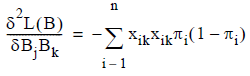where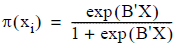Unlike the case with linear regression, confidence intervals are not computed directly on the standard error values, but on something called the odds ratios, described below.

## Odds Ratios and Confidence Intervals

In linear regression, the meaning of each b- coefficient in the model can be thought of as the amount the dependent y variable changes when the corresponding independent x variable changes by 1. Because of the logit transformation, however, the meaning of each b- coefficient in a logistic regression model is not so clear. In a logistic regression model, the increase of an x variable by 1 implies a change in the odds that the outcome y variable will be 1 rather than 0.

Looking back at the formula for the logit response function: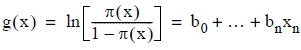it is evident that the response function is actually the log of the odds that the response is 1, where π(x) is the probability that the response is 1 and 1 – π(x) is the probability that the response is 0. Now suppose that one of the x variables, say x j , varies by 1. Then the response function will vary by b j . This can be written as g(x 0 ...x j + 1...x n ) - g(x 0 ...x j ...x n ) = b j . But it could also be written as: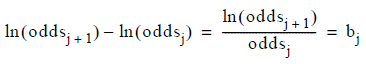Therefore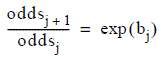the formula for the odds ratio of the coefficient b j . By taking the exponent of a b- coefficient, one gets the odds ratio that is the factor by which the odds change due to a unit increase in x j .

Because this odds ratio is the value that has more meaning, confidence intervals are calculated on odds ratios for each of the coefficients rather than on the coefficients themselves. The confidence interval is computed based on a 95% confidence level and a two-tailed normal distribution.

## Logistic Regression Goodness of fit

In linear regression, one of the key measures associated with goodness of fit is the residual sums of squares RSS. An analogous measure for logistic regression is a statistic sometimes called the deviance. Its value is based on the ratio of the likelihood of a given model to the likelihood of a perfectly fitted or saturated model and is given by D = -2ln(ModelLH / SatModelLH). This can be rewritten D=-2L M + 2L S in terms of the model log likelihood and the saturated model log likelihood. Looking at the data as a set of n independent Bernoulli observations, L S is actually 0, so that D = -2L M . Two models can be contrasted by taking the difference between their deviance values, which leads to a statistic G = D 1 - D 2 = -2(L 1 - L 2 ). This is similar to the numerator in the partial F test in linear regression, the extra sums of squares or ESS mentioned in the section on linear regression.

In order to get an assessment of the utility of the independent model terms taken as a whole, the deviance difference statistic is calculated for the model with a constant term only versus the model with all variables fitted. This statistic is then G = -2(L 0 - L M ). L M is calculated using the log likelihood formula given earlier. L 0 , the log likelihood of the constant only model with n observations is given by: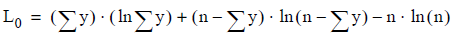G follows a chi-square distribution with “variables minus one” degrees of freedom, and as such provides a probability value to test whether all the x-term coefficients should in fact be zero.

Finally, there are a number of pseudo R-squared values that have been suggested in the literature. These are not truly speaking goodness of fit measures, but can nevertheless be useful in assessing the model. Teradata Warehouse Miner provides one such measure suggested by McFadden as (L 0 - L M ) / L 0 . [Agresti]